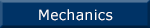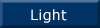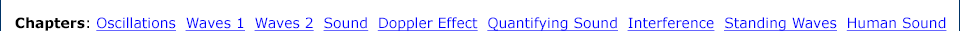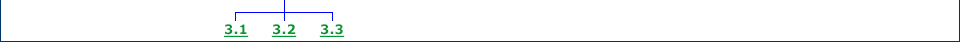Links to related material and teachers' resources The wave equation and wave speed Deriving the wave equation. The sinusoidal solution to the wave equation. The speed of a wave in a stretched string. Experiment: how the wave speed depends on tension.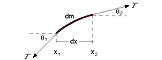Wave power, intensity and radiation Power in a wave. Intensity and power. Radiation in three dimensions. Radiation in two dimension. Reflection in two dimensions.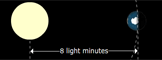Differential equations Methods for solving differential equations. First order with constant coefficients. Second order and simple harmonic motion. Damped and forced oscillation. Partial differential equations: the wave equation.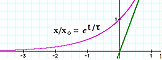Calculus Differentiation: How rapidly does something change? Varying derivatives. Analytical derivatives. Power terms and polynomials. Trigonometric functions. The chain rule. Integration: How do the results of a variable rate add up? Numerical integration. Analytical integration. The log function. Trigonometric functions. The exponential function. Techniques for integration. Partial derivatives.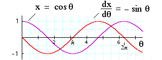Laboratory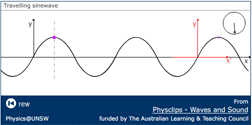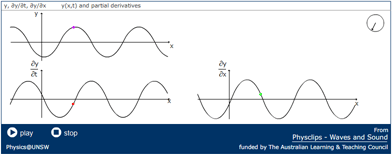Download (mp4 video) Download (original flash file) Download (mp4 video) Download (original flash file)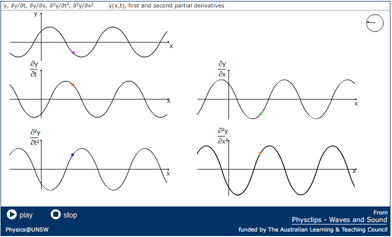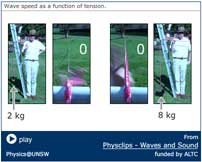Download (mp4 video) Download (original flash file) Download (mp4 video) Download (original flash file)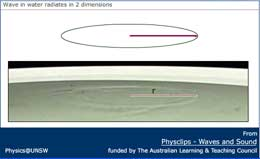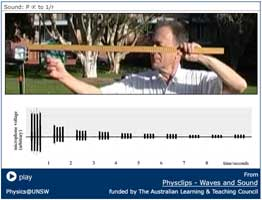Download (mp4 video) Download (original flash file) Download (mp4 video) Download (original flash file)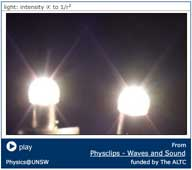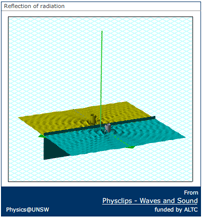Download (mp4 video) Download (original flash file) Download (mp4 video) Download (original flash file)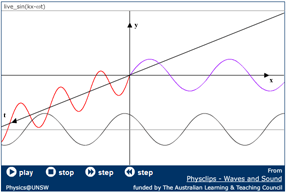Download (mp4 video) Download (original flash file) Download (mp4 video) Download (original flash file)